# CLIJ2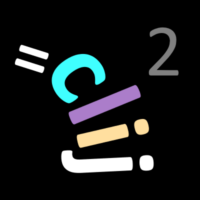GPU accelerated image processing for everyone

CLIJ2 home

# Neighbors of neighbors

Authors: Robert Haase, Daniela Vorkel, April 2020

Source

This macro shows how to apply a filter to values of a graph, considering neighbors of neighbors.

```
// initialize GPU
run("CLIJ2 Macro Extensions", "cl_device=[GeForce RTX 2060 SUPER]");
Ext.CLIJ2_clear();

run("Close All");

```

## The dataset

We start with a list of random point coordinates, draw a spots image and partition the image space between these points.

```
// make a pointlist of random coordinates in the GPU
number_of_points = 200;
number_of_dimensions = 2;
bit_depth = 32;
Ext.CLIJ2_create2D(pointlist, number_of_points, number_of_dimensions, bit_depth);
random_min = 0;
random_max = 400;
seed = getTime();
Ext.CLIJ2_setRandom(pointlist, random_min, random_max, seed);

// draw labelled spots (1, 2, 3, ...) in 2D space
Ext.CLIJ2_pointlistToLabelledSpots(pointlist, spots_image);

// partition the space between points
Ext.CLIJ2_labelVoronoiOctagon(spots_image, label_map);
Ext.CLIJ2_pull(label_map);
run("glasbey_on_dark");
```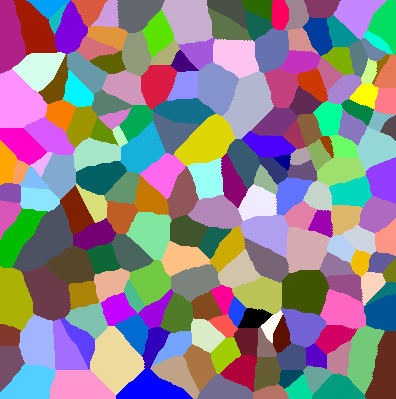Before running operations on neighbors, we need to determine which labels of the label map are neighbors. Furthermore, we can draw the neighborhood relationships as a graph/mesh.

```// determine the touch matrix
Ext.CLIJ2_generateTouchMatrix(label_map, touch_matrix);

// draw mesh
Ext.CLIJ2_touchMatrixToMesh(pointlist, touch_matrix, mesh);
Ext.CLIJ2_pull(mesh);
```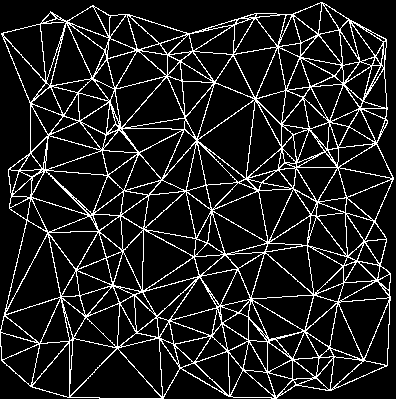## Distribute values in space

We get the center of mass of all objects in our label map and generate vector measurement values which are a bit random, but also differ on the left and right side of the image.

```run("Clear Results");
Ext.CLIJ2_statisticsOfBackgroundAndLabelledPixels(label_map, label_map);
for (i = 0; i < nResults(); i++) {
offset = 80;
if (getResult("MASS_CENTER_X", i) > 200) {
offset = 60;
}
setResult("Measurement", i, offset + random() * 20);
}
Ext.CLIJ2_pushResultsTableColumn(measurement, "Measurement");
run("Clear Results");

```

## Create a parametric image showing measurements in 2D space

```drawResult(label_map, measurement);

function drawResult(label_map, measurement) {
// replace label in the label map with corresponding measurements
Ext.CLIJ2_replaceIntensities(label_map, measurement, parametric_image);
// show the parametric image
Ext.CLIJ2_pull(parametric_image);
setMinAndMax(50, 100);
run("Fire");
}

```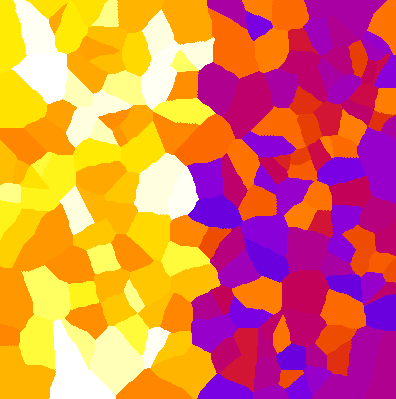## Smoothing between neighbors

We can apply smoothing operations, e.g. the mean filter:

```// determine the mean value of each neighboring nodes
Ext.CLIJ2_meanOfTouchingNeighbors(measurement, touch_matrix, mean_measurement);
drawResult(label_map, mean_measurement);
```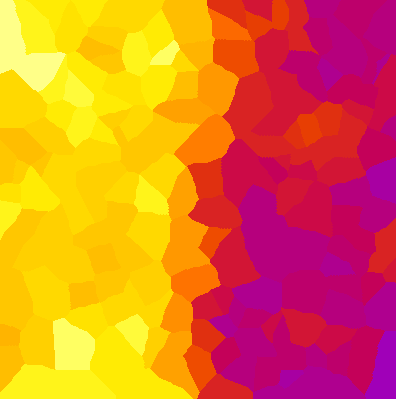To prevent the orange stripe in the center, we use the median filter:

```// determine the median value of each neighboring nodes
Ext.CLIJ2_medianOfTouchingNeighbors(measurement, touch_matrix, median_measurement);
drawResult(label_map, median_measurement);
```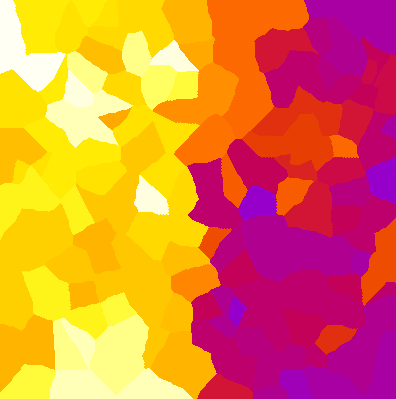Based on that image, we can visualize the border:

```// determine the standard deviation between neighbors
Ext.CLIJ2_standardDeviationOfTouchingNeighbors(median_measurement, touch_matrix, stddev_measurement);
drawResult(label_map, stddev_measurement);
setMinAndMax(0, 10);

```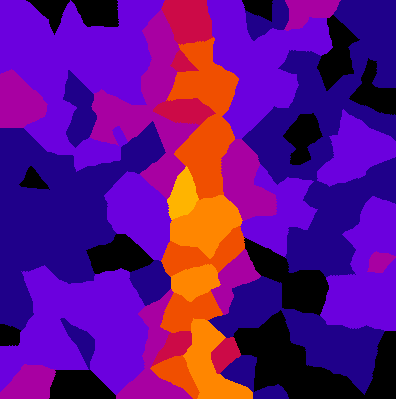## Increasing the radius of operation

Furthermore, we can increase the radius of operation by using `neighborsOfNeighbors();`. When applying the mean filter, one can see a wider orange stripe in the middle of the image.

```
Ext.CLIJ2_neighborsOfNeighbors(touch_matrix, neighbor_matrix);
Ext.CLIJ2_meanOfTouchingNeighbors(measurement, neighbor_matrix, mean_measurement);
drawResult(label_map, mean_measurement);

```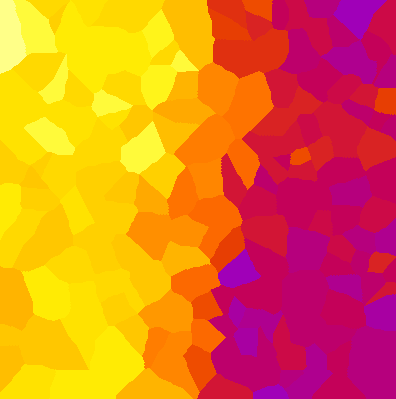At the end of the macro, clean up:

```Ext.CLIJ2_clear();

```